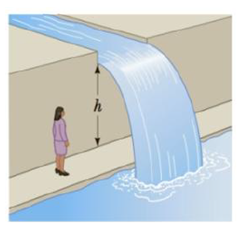Chapter 3, Problem 54AP

Chapter
Section
Textbook Problem

A landscape architect is planning an artificial waterfall in a city park. Water flowing at 0.750 m/s leaves the end of a horizontal channel at the top of a vertical wall h = 2.35 m high and falls into a pool (Fig. P3.54). (a) How far from the wall will the water land? Will the space behind the waterfall be wide enough for a pedestrian walkway? (b) To sell her plan to the city council, the architect wants to build a model to standard scale, one-twelfth actual size. How fast should the water flow in the channel in the model?Figure P3.54

(a)

To determine
The distance from the wall the water will land. If the space behind the waterfall be wide enough for the pedestrian walkway.

Explanation

The water is falling is free fall. So, the elapsed time when the droplet reaches the pool is,

tP=2hg

Here,

h is the height from where the water falls

g is the acceleration due to gravity

The distance from the wall where the water lands is,

R=xmax=v0xtP=v0x2hg

Here,

v0x is the horizontal component of the initial velocity

Substitute 0.75m/s for v0 , 2

(b)

To determine
The speed of the water flow in the channel in the model.

Still sussing out bartleby?

Check out a sample textbook solution.

See a sample solution

The Solution to Your Study Problems

Bartleby provides explanations to thousands of textbook problems written by our experts, many with advanced degrees!

Get Started